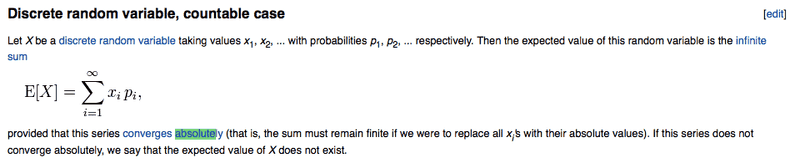# Expected Value of Positive-Valued RV

## Homework Statement

Prove that if X is a positive-valued RV, then E(X^k) ≥ E(X)^k for all k≥1

## The Attempt at a Solution

Why do I feel like this is a counter-example:

X = {1,2,4,8,16,...} (A positive-valued RV)
m(X) = {1/2,1/4,1/16,1/32,...} (A distribution function that sums to one)

Yet clearly,

$$E[X] = \sum _{k=1} ^{∞} \frac{1}{2^k} {2}^{k-1} = \frac{1}{2} + \frac{1}{2} + \frac{1}{2} + \frac{1}{2} + ... = ∞$$

So the expected value diverges (ie. doesn't exist). So I can't do the proof because for this RV, the expectation DNE.

Last edited:

Ray Vickson
Science Advisor
Homework Helper
Dearly Missed

## Homework Statement

Prove that if X is a positive-valued RV, then E(X^k) ≥ E(X)^k for all k≥1

## The Attempt at a Solution

Why do I feel like this is a counter-example:

X = {1,2,4,8,16,...} (A positive-valued RV)
m(X) = {1/2,1/4,1/16,1/32,...} (A distribution function that sums to one)

Yet clearly,

$$E[X] = \sum _{k=1} ^{∞} \frac{1}{2^k} {2}^{k-1} = \frac{1}{2} + \frac{1}{2} + \frac{1}{2} + \frac{1}{2} + ... = ∞$$

So the expected value diverges (ie. doesn't exist). So I can't do the proof because for this RV, the expectation DNE.

This is also an allowable case if you accept that ∞ ≥ ∞, and that EX = ∞ implies EX^k = ∞ for any k > 1.

However, in the case that EX < ∞ and EX^k < ∞, can you do the proof then?

RGV

## Homework Statement

Prove that if X is a positive-valued RV, then E(X^k) ≥ E(X)^k for all k≥1

## The Attempt at a Solution

Why do I feel like this is a counter-example:

X = {1,2,4,8,16,...} (A positive-valued RV)
m(X) = {1/2,1/4,1/16,1/32,...} (A distribution function that sums to one)

Yet clearly,

$$E[X] = \sum _{k=1} ^{∞} \frac{1}{2^k} {2}^{k-1} = \frac{1}{2} + \frac{1}{2} + \frac{1}{2} + \frac{1}{2} + ... = ∞$$

So the expected value diverges (ie. doesn't exist). So I can't do the proof because for this RV, the expectation DNE.

Why can't you? Both $E[X^2]$ and $E[X]^2$ are infinite, right?

So I went and got this from Wikipedia:I wouldn't have much of a problem accepting ∞ ≥ ∞ if I thought that the expectation of such an RV even existed. Since the expectation diverges in my example, it seems meaningless to me to discuss comparisons of its nonexistent expected value.

Simon Bridge
Science Advisor
Homework Helper
I suspect you have over-complicated things... surely it is not true, in general, that the sum of powers is equal to the power of the sum - especially if all elements in the sum are positive? Consider if X is drawn from a set of two values for instance...

That's if I've read it correctly that you have to show:
$$E(X^k)=\frac{1}{N}\sum_{i=1}^{N}(x_i)^k = \bigg ( \frac{1}{N}\sum_{i=1}^{N}x_i \bigg )^k = \bigg ( E(X) \bigg ) ^k$$

Ray Vickson
Science Advisor
Homework Helper
Dearly Missed
So I went and got this from Wikipedia:I wouldn't have much of a problem accepting ∞ ≥ ∞ if I thought that the expectation of such an RV even existed. Since the expectation diverges in my example, it seems meaningless to me to discuss comparisons of its nonexistent expected value.

Opinions vary, and not all books would agree with that Wiki article. Of course, the expectation does not exist (as a real number), but in such a case we sometimes write EX = ∞ and pretend that ∞ is in an extended real number field.

Note that there are essentially two kinds of "EX does not exist": (1) EX = ∞; and (2) for X in all of ℝ, with X = X+ - X-, with X± ≥ 0 and EX+ = EX- = ∞ (that is, EX would be of the form ∞ - ∞).

Anyway, if you don't like this, add finiteness statements to the hypotheses. That still leaves you with something to prove.

RGV

I suspect you have over-complicated things... surely it is not true, in general, that the sum of powers is equal to the power of the sum - especially if all elements in the sum are positive? Consider if X is drawn from a set of two values for instance...

That's if I've read it correctly that you have to show:
$$E(X^k)=\frac{1}{N}\sum_{i=1}^{N}(x_i)^k = \bigg ( \frac{1}{N}\sum_{i=1}^{N}x_i \bigg )^k = \bigg ( E(X) \bigg ) ^k$$
I don't think that's what I'm trying to show. I want to show a weak inequality, as opposed to a strict equality. Also, you seem to have assumed a uniform pdf (ie. the 1/N), which does not seem a fair assumption to me.

Opinions vary, and not all books would agree with that Wiki article. Of course, the expectation does not exist (as a real number), but in such a case we sometimes write EX = ∞ and pretend that ∞ is in an extended real number field.

Note that there are essentially two kinds of "EX does not exist": (1) EX = ∞; and (2) for X in all of ℝ, with X = X+ - X-, with X± ≥ 0 and EX+ = EX- = ∞ (that is, EX would be of the form ∞ - ∞).

Anyway, if you don't like this, add finiteness statements to the hypotheses. That still leaves you with something to prove.

RGV
Yes, I think I'll have to add convergence hypotheses. In which case, the proof is immediate from Jensen's Inequality.

Ray Vickson
Science Advisor
Homework Helper
Dearly Missed
Yes, I think I'll have to add convergence hypotheses. In which case, the proof is immediate from Jensen's Inequality.

I think you also need to show that EX = ∞ implies EX^k =∞ for all k > 1; that is, if EX does not exist then neither does EX^k.

RGV